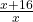## Find a number such that if 16 is added to it, the ratio of the result to the original number is 4:3

Question

Find a number such that if 16 is added to it, the ratio of the result to the original number is 4:3

in progress 0
1 month 2021-08-02T10:12:11+00:00 1 Answers 5 views 0

48

Step-by-step explanation:

let the number be x and x + 16 the result of adding 16 to it, then

the ratio of the result to the original is=( cross- multiply )

4x = 3(x + 16)  = 3x + 48 ( subtract 3x from both sides )

x = 48